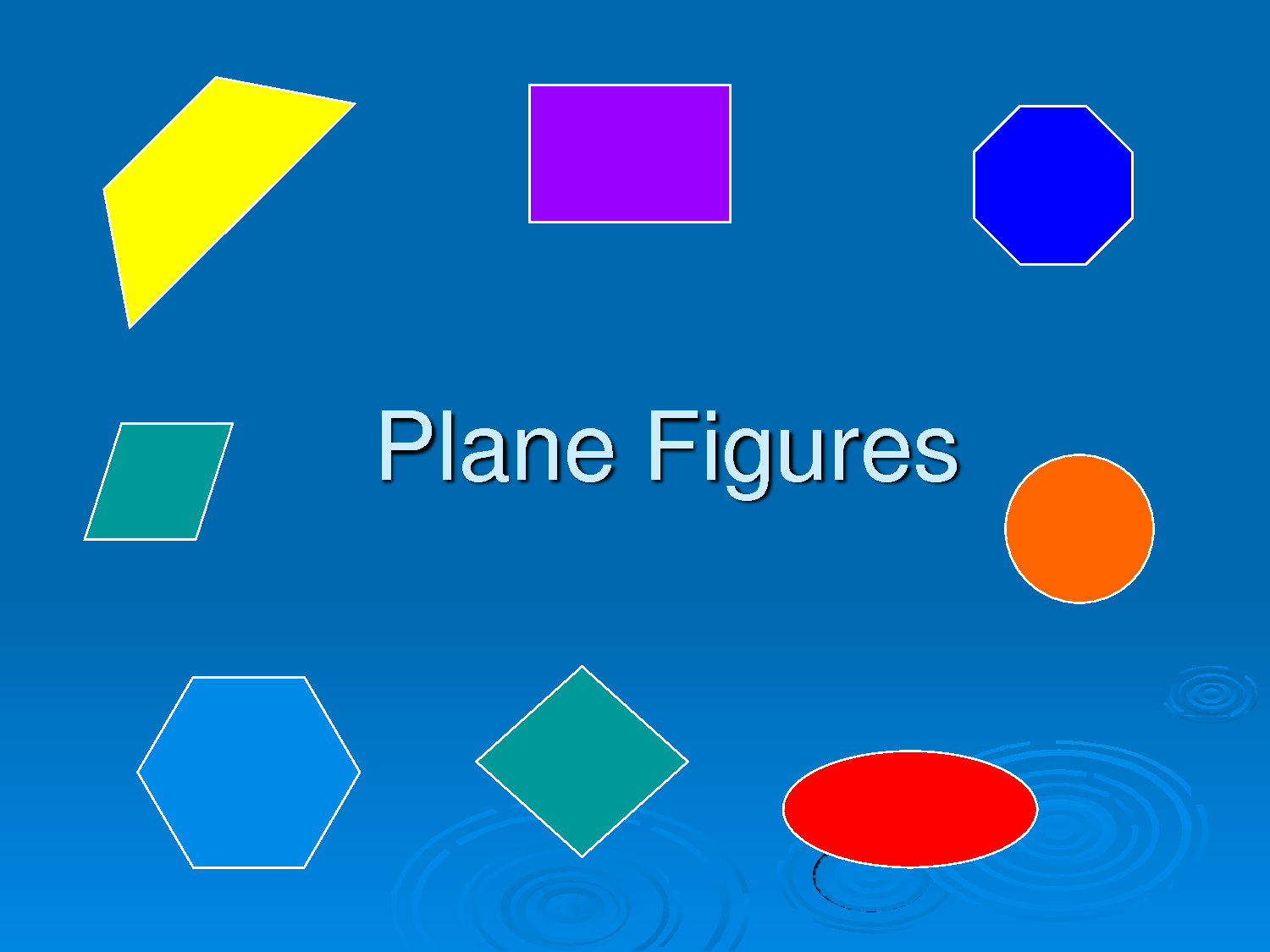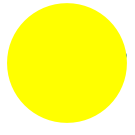# GEOMETRY PLANE SHAPES

Welcome to class!

In today’s class, we will be talking about geometry plane shapes. Enjoy the class!

### GEOMETRY PLANE SHAPES1. Types of plain shapes and their properties
2. Similarities and differences between square, rectangles, triangles, trapeziums, parallelograms, and circles

##The circle is a shape that can be made by tracing a curve that is always the same distance from a point that we call the centre. The distance around a circle is called the circumference of the circle.

##The triangle is a shape that is formed by 3 straight lines that are called sides. There are different ways of classifying triangles, according to their sides or angles.

1. According to their angles:
• Right triangle: the largest of the 3 angles is a right angle.
• Acute Triangle: the largest of the 3 angles is an acute angle (less than 90 degrees).
• Obtuse Triangle: the largest of the 3 angles is an obtuse angle (more than 90 degrees).
1. According to their sides:
• Equilateral Triangle: all 3 sides are the same length.
• Isosceles Triangle: it has 2 (or more) sides that are of equal length. (An equilateral triangle is also isosceles.)
• Scalene Triangle: no 2 sides are of equal measure.

##The rectangle is a shape that has 4 sides. The distinguishing characteristic of a rectangle is that all 4 angles measure 90 degrees.

##The rhombus is a shape formed by 4 straight lines. Its 4 sides measure the same length but, unlike the rectangle, any of all 4 angles measure 90 degrees.

##The square is a type of rectangle, but also a type of rhombus. It has characteristics of both of these. That is to say, all 4 angles are right angles, and all 4 sides are equal in length.

##The trapezoid also has 4 sides. It has two sides that are parallel but the other 2 are not.

• #### Trapezium and Its Properties:

A trapezium or a trapezoid is a quadrilateral with a pair of parallel sides. A parallelogram may also be called a trapezoid as it has two parallel sides. The pair of parallel sides is called the base while the non-parallel sides are called the legs of the trapezoid. The line segment that connects the midpoints of the legs of a trapezoid is called the mid-segment.

#One special kind of polygons is called a parallelogram. It is a quadrilateral where both pairs of opposite sides are parallel.

In our next class, we will be talking more about Geometry Plane Shapes.  We hope you enjoyed the class.

Should you have any further question, feel free to ask in the comment section below and trust us to respond as soon as possible.

How Can We Make ClassNotesNG Better? - CLICK to Tell Us Now!